# Cone

Go back to  'Geometry'

 1 Introduction to Cone 2 Definition of Cone 3 Types of Cone 4 Examples of Cone-shaped Objects 5 Properties of Cone 6 Challenging Question on Cone 7 Surface Area of Cone 8 Volume of Cone 9 Important Notes on Cone 10 Solved Examples on Cone 11 Practice Questions on Cone 12 Maths Olympiad Sample Papers 13 Frequently Asked Questions (FAQs)

We at Cuemath believe that Math is a life skill. Our Math Experts focus on the “Why” behind the “What.” Students can explore from a huge range of interactive worksheets, visuals, simulations, practice tests, and more to understand a concept in depth.

Book a FREE trial class today! and experience Cuemath’s LIVE Online Class with your child.

## Introduction to Cone

The term “cone” is derived from the Greek word "konos" which means "a wedge" or "a peak".

The first thing that comes to our mind when we think of a cone is an ice-cream cone.

Do you know who made the first ice-cream cone?

The first ice-cream cone was made by Marchiony from Italy in the late 1800s.

He invented it in New York City and was granted a patent for the cone a few years later.

Interesting, isn't it?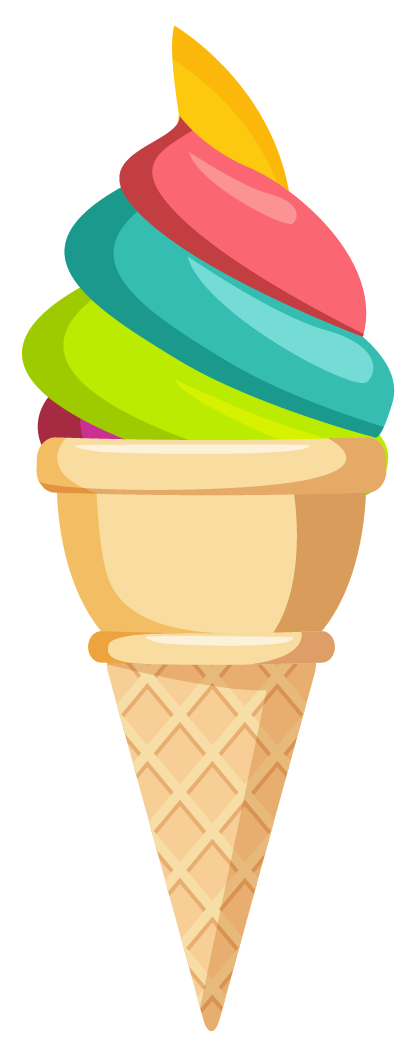Similar to the ice-cream cone, there are many objects around us that have the shape of a cone.

## Definition of Cone

A cone is a three-dimensional shape that has a flat surface at one end and a curved surface pointing outward called apex at the other end.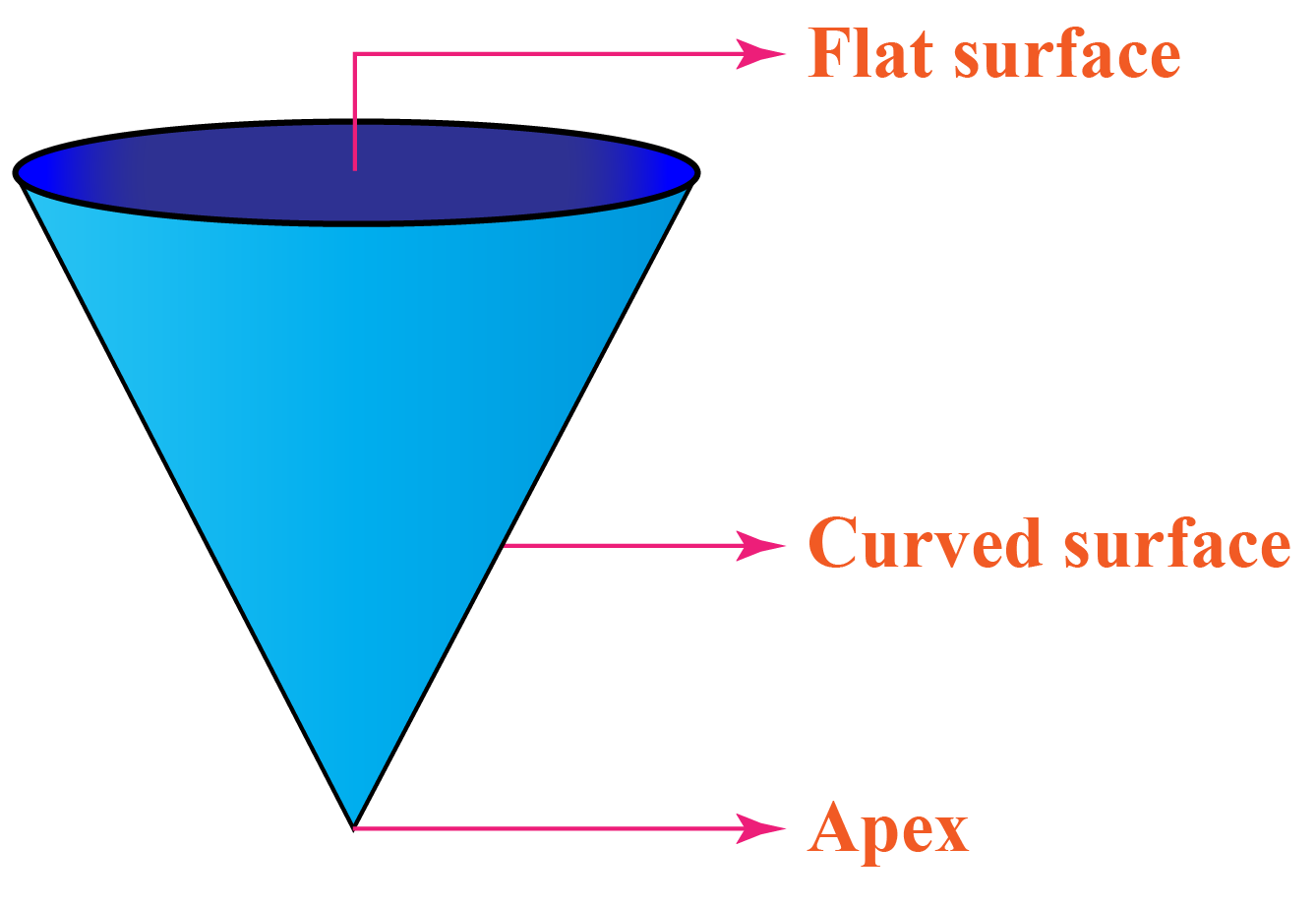A cone has one curved surface, one vertex called apex and no edges.

### Parameters of Cone

In general, a cone shape consists of three parameters.

1. Height (h)
2. The radius of the base (r)
3. Slant height (s)

Height (h): It is the perpendicular distance from the vertex to the flat plane containing the base.

Slant Height (s): It is the distance between the vertex and the boundary of the base of a cone. It is denoted by $$s$$ or $$l$$.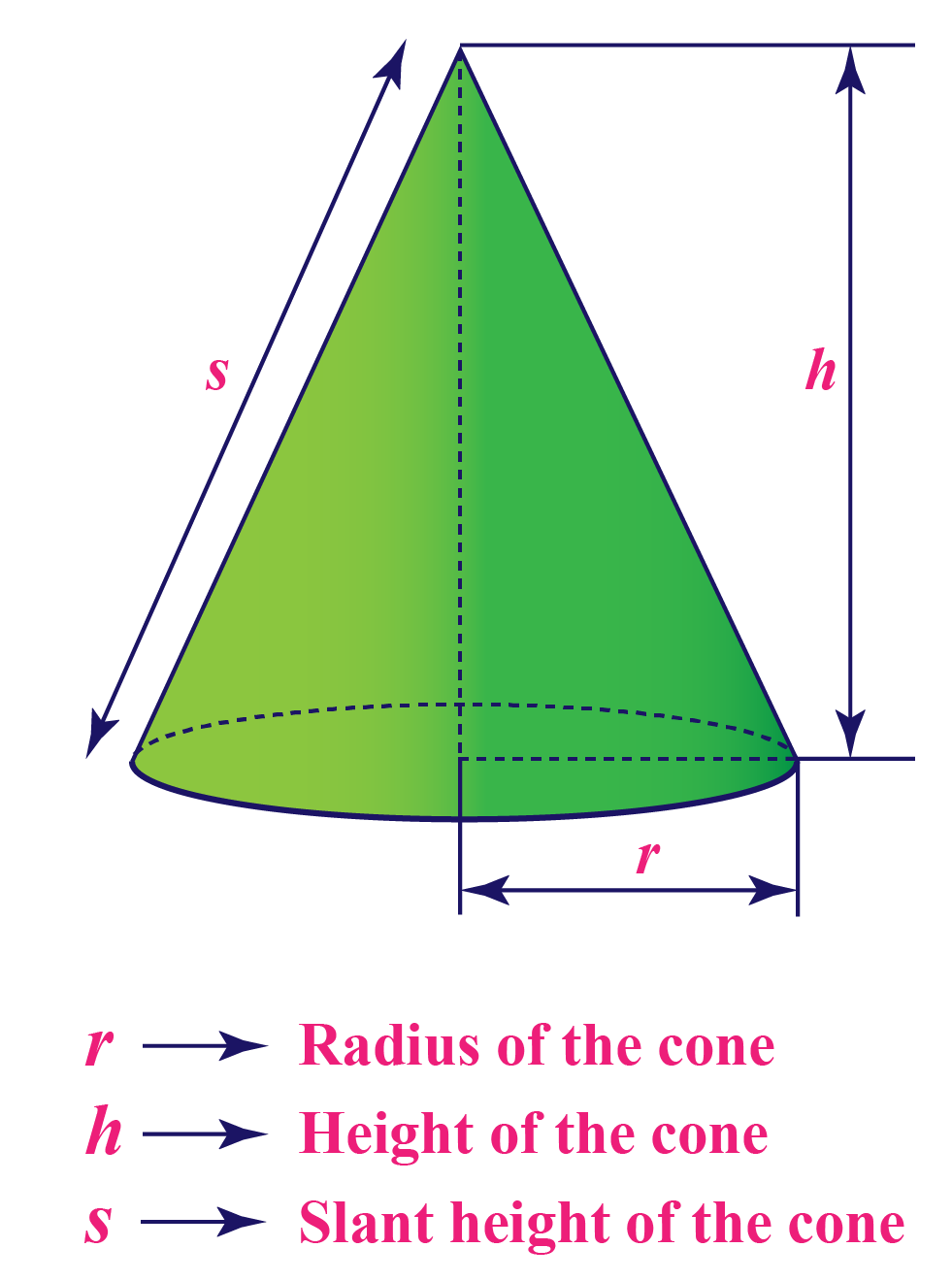## Types of Cones

Based on the orientation of the vertex on to the circular base, cones can be categorized into:

• Right Circular Cone
• Oblique Cone

### Right Circular Cone

A right circular cone has the apex perpendicular to the base, which means that the perpendicular line falls exactly on the center of the circular base of the cone.

In the right circular cone, the vertex is at the same distance from every point on the boundary of the base.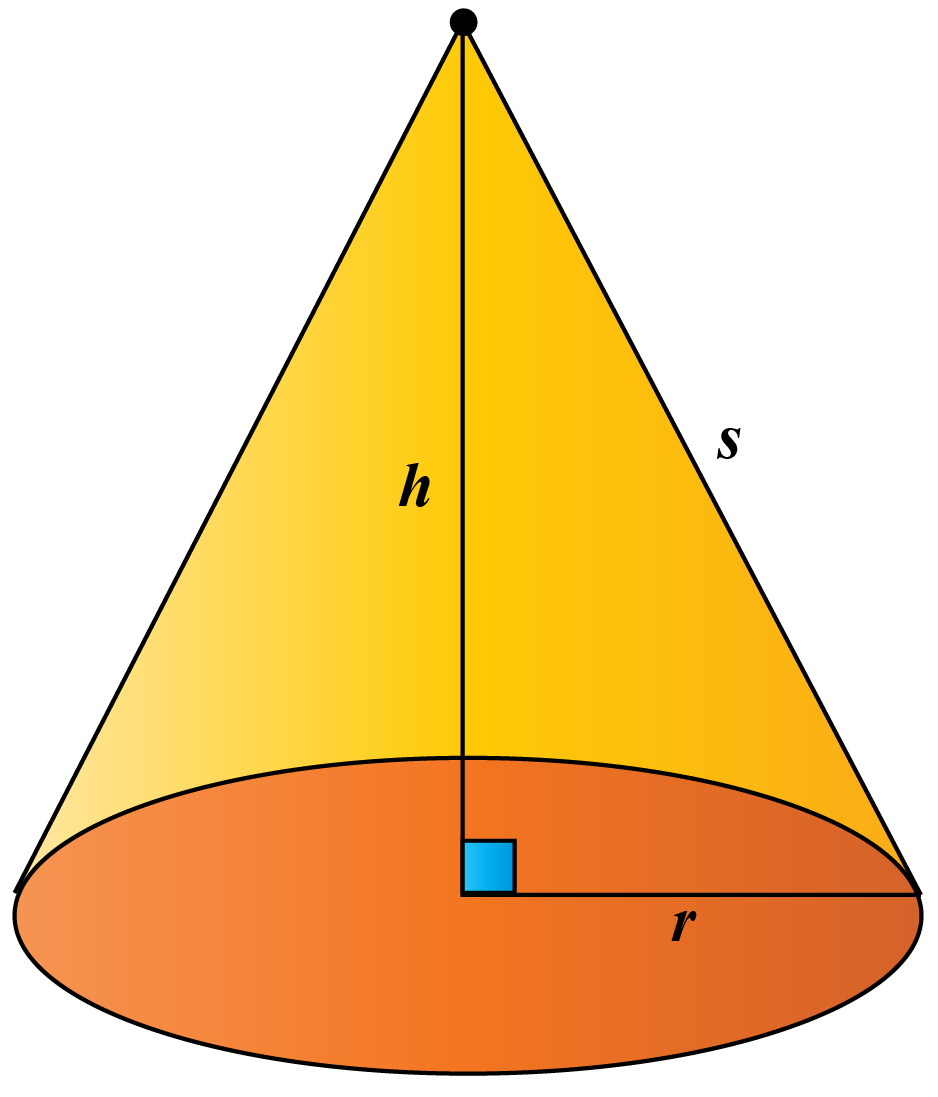### Oblique Cone

The cone in which the position of the apex is not perpendicular to the center of the base.

The oblique cone has the vertex which is not centered over the base and it leans over.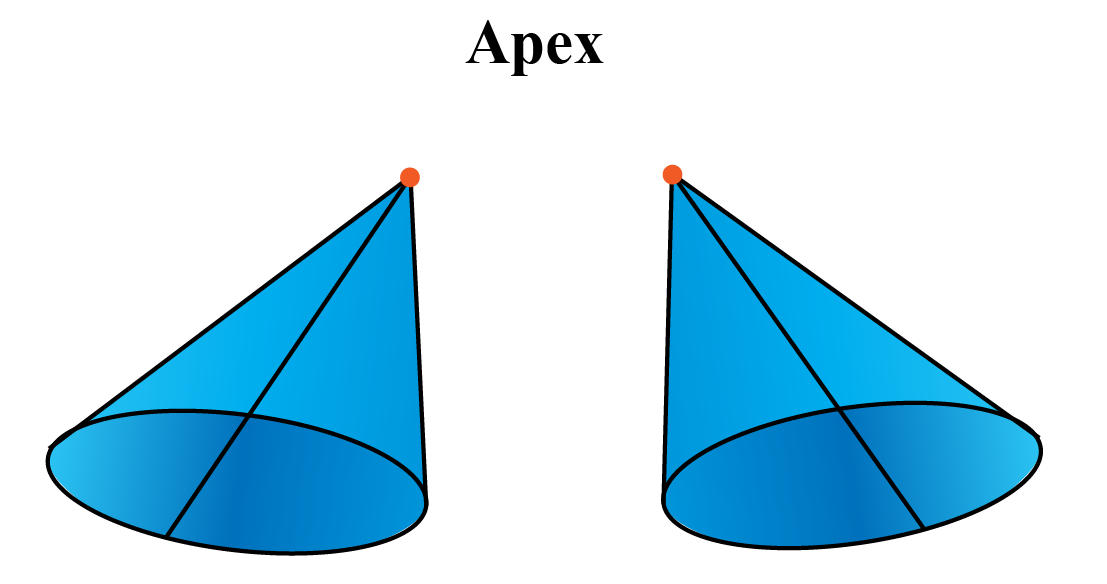## Examples of Cone-Shaped Objects

We see several cone-shaped objects in our daily life.

Few real-life examples of objects with cone shape are as follows: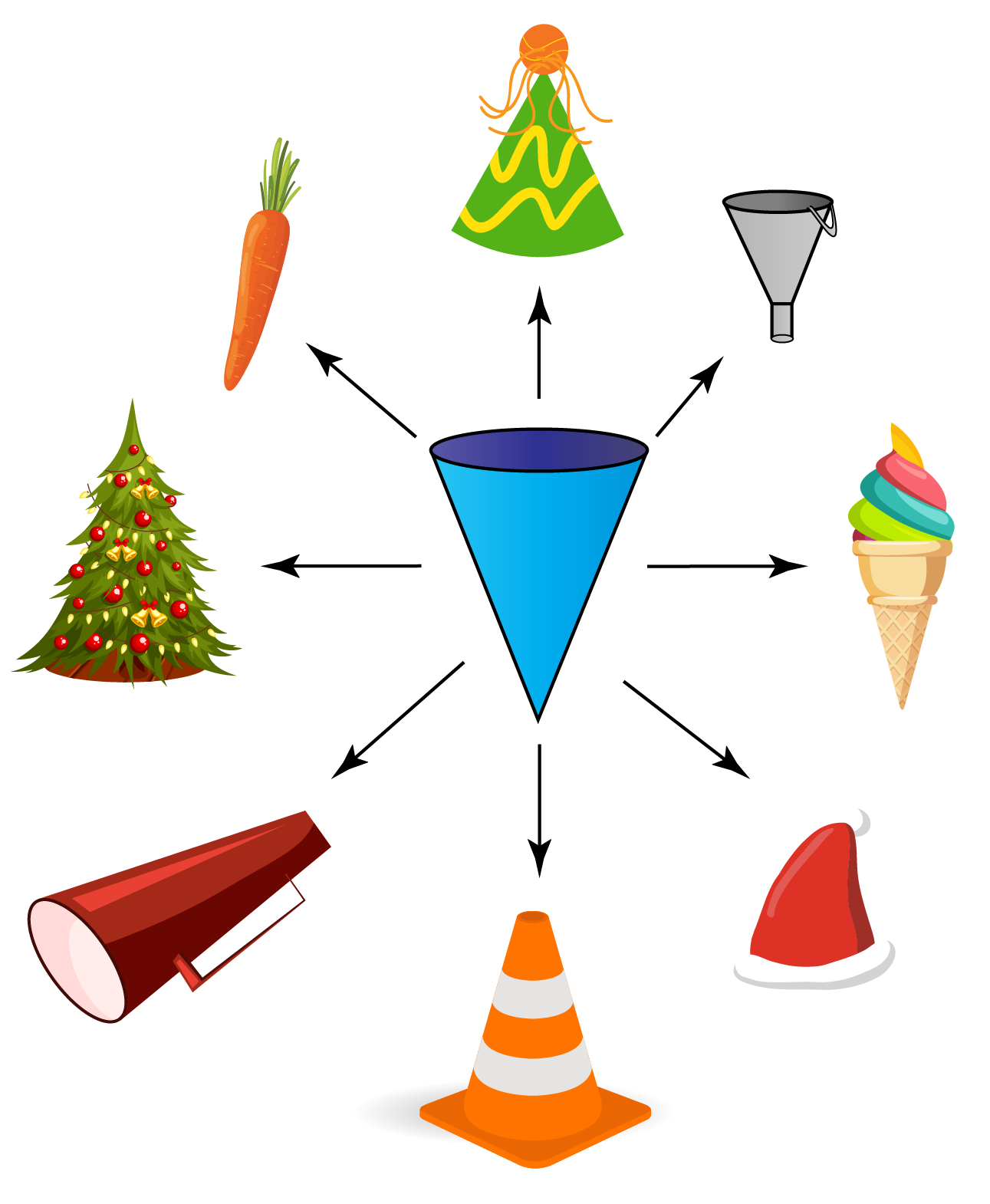## Properties of Cone

A cone has some properties that differentiates it from other solid shapes.

1. A cone has no edges.
2. A cone has only one apex.
3. The volume of a cone is $$\frac{1}{3}\pi r^2 h$$.
4. The total surface area of a cone is $$\pi r (l+r)$$
5. The slant height of a cone is $$\sqrt{r^2 + h ^2}$$.

### Cone Formula

The cone formula refers to the relation between height, radius, and slant height of a cone.

This formula applies the Pythagoras Theorem.

 $$s = \sqrt{h^2 + r^2}$$

When the radius $$r$$, height $$h$$ and slant height $$s$$ of a cone are given, then the following formulas apply.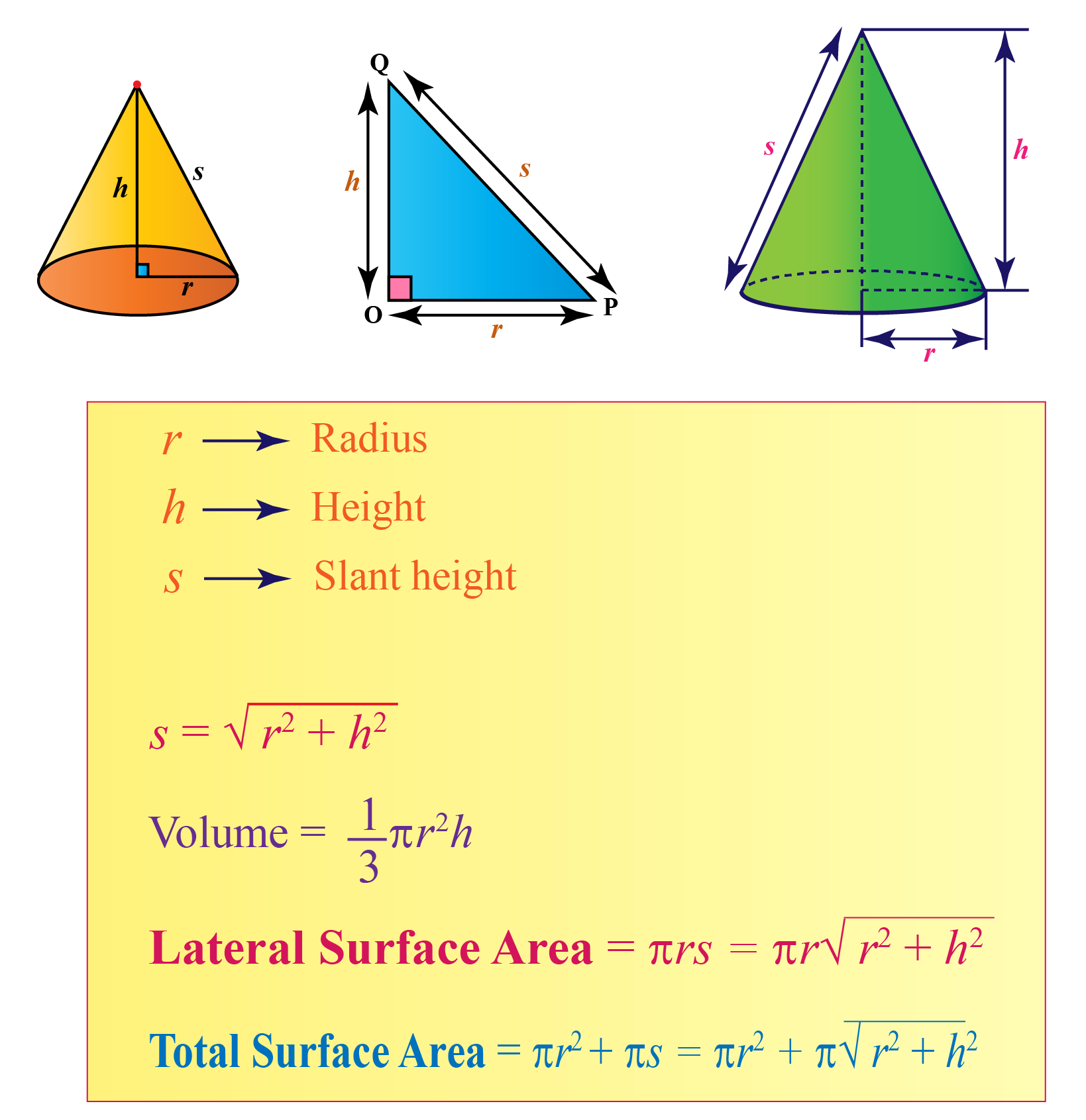### What is a conic section?

In mathematics, a conic section (or simply conic) is a curve obtained as the intersection of the surface of a cone with a plane.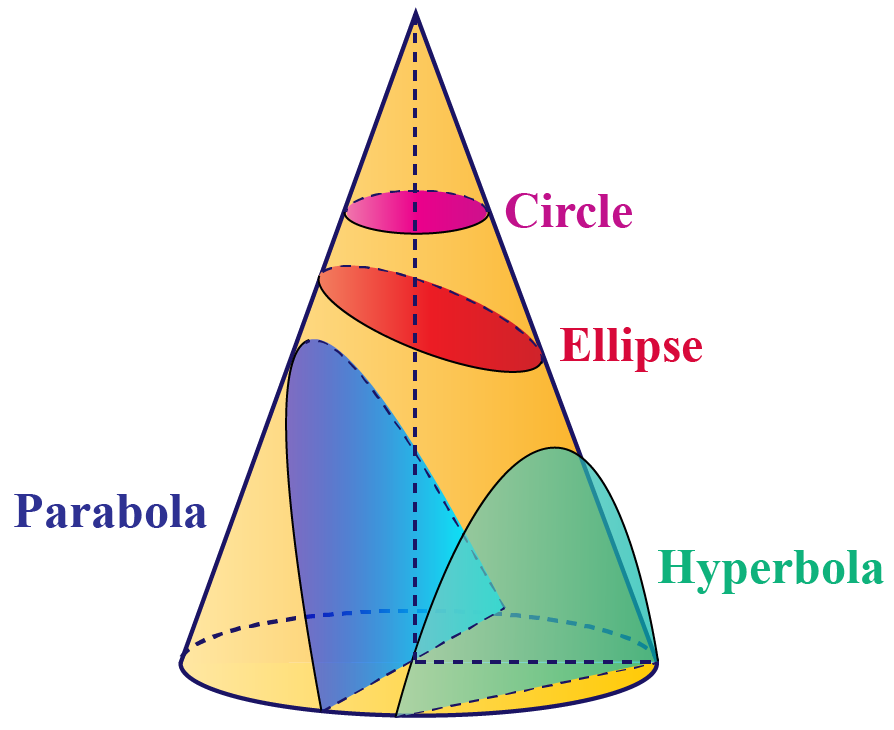The three types of conic sections are:

• hyperbola
• parabola
• ellipse
• circle (a special case of the ellipse)Challenging Questions
1. The icing bags Tina uses to decorate cakes are cone shaped.

Each icing bag has a radius of 4 cm and a height of 11 cm.

If Tina needs to use three bags of icing to decorate a cake, how much icing does she need to buy? (use $$\pi=3.14$$)

## Surface area of Cone

The total surface area of a cone consists of two parts:

• The curved surface area
• The flat surface area (of the base)

The flat surface area is simply $$\pi {r^2}$$ since the base is circular in shape.

### What is the curved surface area?

To determine the curved surface area, visualize opening the cone and rolling it out.

What is the shape that is formed?

It is a circular sector.

Let's try this activity to see if this is true.

Cut out a circular sector on a paper.

Then, fold/roll the paper so that the two straight edges meet each other.

You can observe that the shape formed is that of a cone.

Note: The radius $$R$$ of the circular sector is the slant height of the cone.

Denote the slant height by $$s$$.

Observe that $$s= \sqrt {{r^2} + {h^2}}$$.

The arc length $$L$$ of the circular sector is the circumference of the base of the cone, which is $$2\pi r$$.

Now, the area of the circular sector is:

$$\pi\:r\:s$$

where $$s$$ is the slant height

so, the total surface area of the cone is the sum of curved surface area and flat surface area

 $$T\:S\:A= \pi {r^2} + \pi r\:s$$

Let's summarize the cone formulas.

Flat SA= $$\pi {r^2}$$

Curved SA = $$\pi r\:s = \pi r\sqrt {{r^2} + {h^2}}$$

Total SA = $$\pi {r^2} + \pi r\:s$$

## Volume of Cone

The volume of a cone can be equalized to one-third parts of the volume of a cylinder.

We know that the volume of any three-dimensional object,

Volume = Base Area X Height

Volume of cylinder = $$\pi r^2h$$
Volume of cone     = $$\frac{1}{3}(volume\: of\: cylinder)$$= $$\frac{1}{3}\pi\:r^2h$$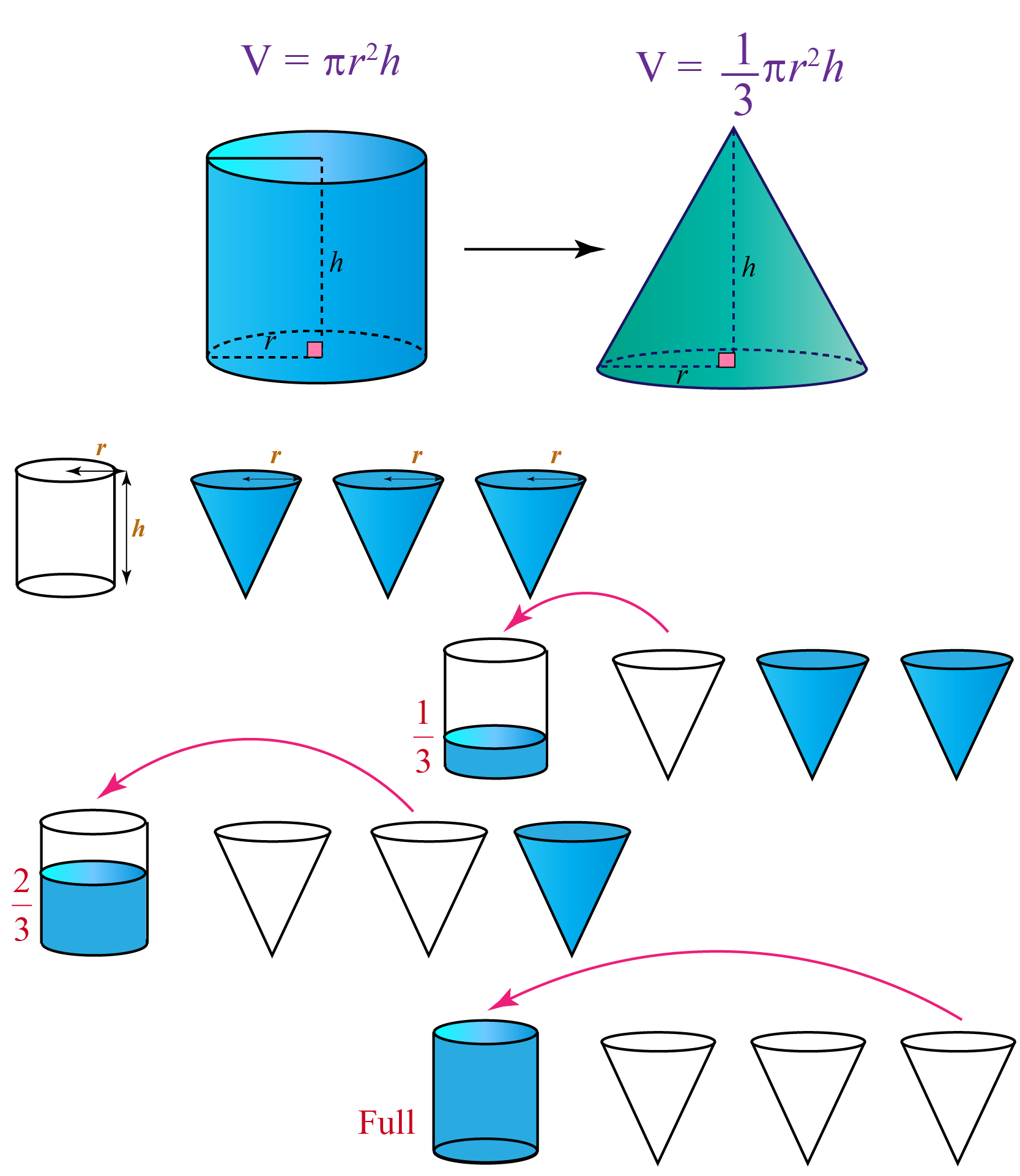$$V=\frac{1}{3} \pi r^2h$$

In the stimulation below you can observe that as the radius and height increase, the volume of the cone is being calculated using the formula written in it.

Help your child score higher with Cuemath’s proprietary FREE Diagnostic Test. Get access to detailed reports, customized learning plans, and a FREE counseling session. Attempt the test now.

## Solved Examples on Cone

 Example 1

For her birthday, Hasmitha wanted to make birthday caps in the form of right circular cones for all her friends.

She made 10 cones of base radius 7 cm and height 24 cm each.

Find the area of the sheet required to make 10 such caps for her friends.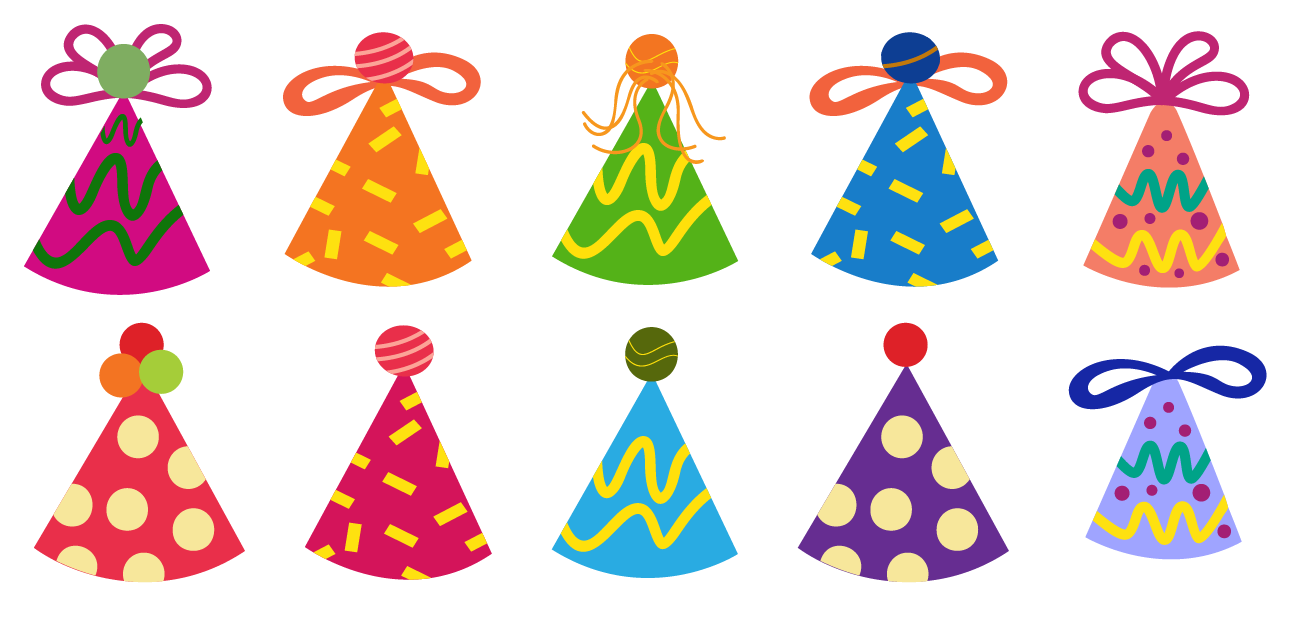Solution:

Given that,

The radius of the cone is, $$r = 7\: cm$$

The height of the cone is, $$h = 24\:cm$$

Slant height will be $s = \sqrt{r^2 + h^2}$

Substituting values of radius and height,

$$\sqrt{7^2 + 24^2}$$

$$\sqrt{49+576}$$

$$\sqrt{625}$$

$$s = 25\:cm$$

Area of the sheet required for 1 cap  = CSA of cone $$=\pi r \times s$$
$$=\frac{22}{7}\times 7\times 25$$
$$= 22\times 25$$
$$= 550\: cm^2$$

Area of sheet required for 10 such caps $= 10 \times 550 = 5500\: cm^2$

 $$\therefore$$ $$5500\:cm^2$$ of the sheet is required
 Example 2

Justin found an hourglass with two cones meeting at their vertex.

The sand in the upper cone falls to the base of the lower cone.

The cones have a radius of 10 mm and a height of 24 mm.

The sand falls at a rate of $$50\: mm^3/sec$$.

How long does it take for the sand to completely drain to the lower cone?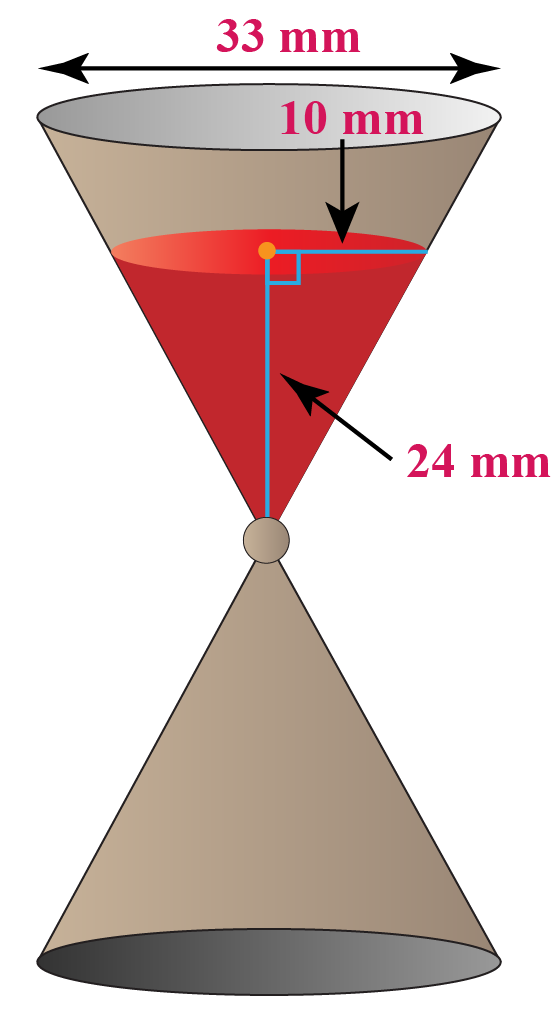Solution:

Given that

Height = 24 mm

It is clear that, the volume of the cone = volume of sand in the cone.

Hence,

$V = \frac{1}{3}\pi r^2h$

$V= \frac{1}{3}\pi(10^2)\times 24$

$V = 800\pi$

$V= 2512\:mm^3$

Thus, the volume of the sand is about $$2512\:mm^3$$.

To find the time, we will substitute the values for volume and the rate at which the sand is falling in this formula.

$T = V\times \frac{1}{R}$

$T = 2512 \times \frac{1}{50}$

$T= 50.24\:sec$

Therefore, the time taken is $$50.24\:sec$$ for the sand to fall completely into the lower cone.

 $$\therefore$$ $$T= 50.24\:sec$$
 Example 3

Harsha found a cone-shaped object like in the figure with height 100 cm and diameter 60 cm on the side of a footpath where work was under progress.

What would be the slant height of that object?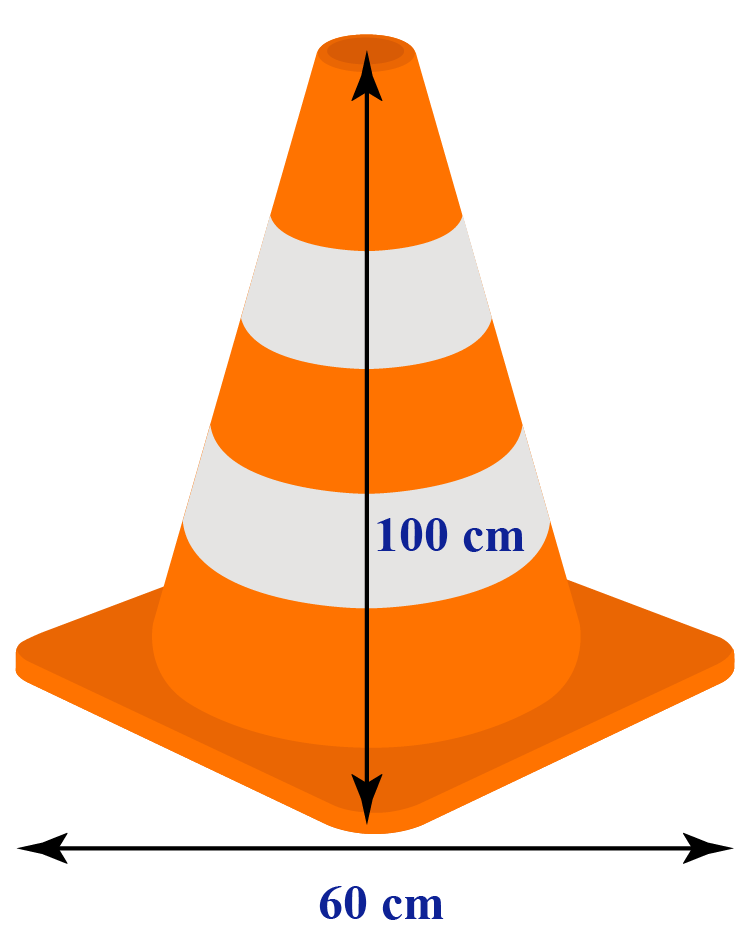Solution:

Given that

height , $$h = 100\: cm$$

Radius, $$r =\frac{60}{2}\:cm = 30\: cm$$

Slant height, $$s = \sqrt{r^2 + h^2}$$

$s = \sqrt { 30^2 + 100^2}$

$s = \sqrt {10900}$

$s = 104.4\: cm$

 $$\therefore$$ the slant height of the traffic cone is 104.4 cm.
 Example 4

Sandy has a cone of volume $$36\:cm^3$$.

Can you find the height of that cone if the diameter is 1.8 cm?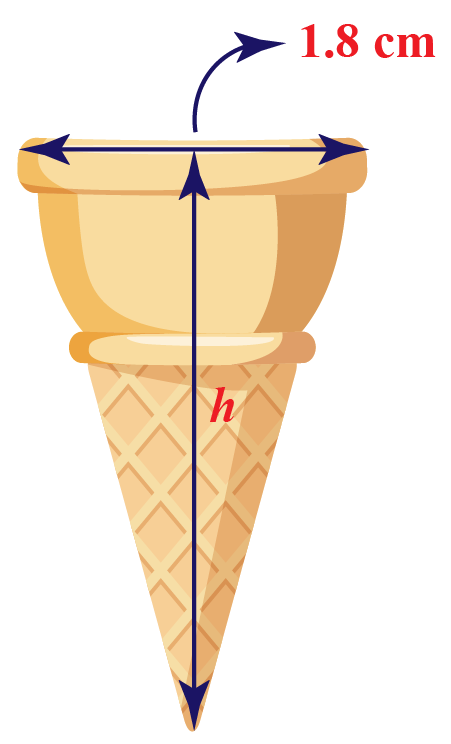Solution:

Given,

The diameter of the cone is 1.8 cm

then the radius of the cone will be \begin{align}\frac{d}{2}\end{align} = \begin{align}\frac{1.8}{2}\end{align} = \begin{align}0.9\end{align}

The volume of the cone is $$36\:cm^3$$

$V = \frac{1}{3}\pi r^2\:h$

$36 = \frac{1}{3}\times \frac{22}{7}\times (0.9)^2\times h$

$h= 38.19\:cm$

 $$\therefore$$ Height of the cone is 38.19 cm
 Example 5

What would be the radius of the cone, if the perpendicular height is 8 cm and the slant height is 10 cm?

Solution:

Given that,

The perpendicular height of the cone = 8 cm

The slant height of the cone               = 10 cm

Using the slant height formula, $s = \sqrt{r^2 + h^2}$

substituting those values we get,

$$10 = \sqrt{r^2 + 8^2}$$

$$100 = r^2 + 64$$

$$r = \sqrt{100 - 64}$$

$$r = 6\:cm$$

 $$\therefore$$ Radius of the cone is 6 cm

Want to understand the “Why” behind the “What”? Explore Cone with our Math Experts in Cuemath’s LIVE, Personalised and Interactive Online Classes.

Make your kid a Math Expert, Book a FREE trial class today!

## Practice Questions on Cone

Here are a few activities for you to practice. Select/Type your answer and click the "Check Answer" button to see the result.

IMO (International Maths Olympiad) is a competitive exam in Mathematics conducted annually for school students. It encourages children to develop their math solving skills from a competition perspective.

## 1. How many edges does a cone have?

A cone has zero edges.

## 2. Is cone a pyramid?

A pyramid typically has a rectangular base and flat, triangular faces whereas a cone has a circular base and a curved face with an apex.

A pyramid can be a cone if its polygon base is circular and if it has a closed curve to give it a cone shape.

## 3. Does a cone have a vertex?

A cone has only one apex or vertex point.

## 4. What is the formula of volume of the cone?

The formula of the volume of the cone is , V = $$\frac{1}{3}\pi r^2\:h$$

More Important Topics
Numbers
Algebra
Geometry
Measurement
Money
Data
Trigonometry
Calculus